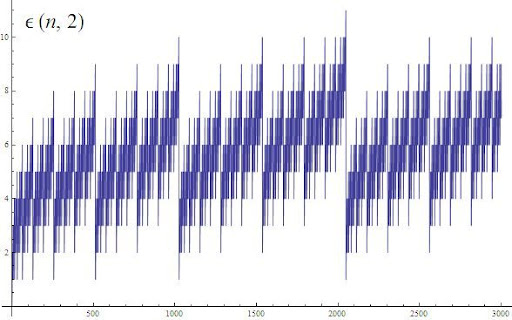## Friday, 18 December 2009

### NOTES ON LEGENDRE'S FORMULA

MORE FACTS ABOUT PRIME FACTORIZATION OF FACTORIALS.

In the previous post, we introduced these functions, just as a small trick to calculate the limit we were looking for, but unlikely of what they seem to be, they are less artificial than expected.

Legendre´s formula for the exponent of p in the prime factorization of n!:

$latex \displaystyle\alpha(n,p)=\sum _{i=1}^{\lfloor log_p(n)\rfloor}{ \bigg\lfloor\frac{n}{p^i} \bigg\rfloor = \sum _{i=1}^{\infty} { \bigg\lfloor\frac{n}{p^i} \bigg\rfloor$

Integer Approximation for the Legendre's formula:

$latex \displaystyle \alpha^{*}(n,p)=\bigg\lfloor \frac{n}{p-1}\bigg\rfloor = \bigg\lfloor\sum _{i=1}^{\infty}{\frac{n}{p^i}}\bigg\rfloor$

The diference between one function and its approximation is the error function.

Error Function for the Legendre's formula:

$latex \displaystyle \epsilon(n,p)=|\alpha^{*}(n,p) - \alpha(n,p) |= \alpha^{*}(n,p) - \alpha(n,p)$

$latex \displaystyle \epsilon(n,p)= \bigg\lfloor\sum _{i=1}^{\infty}{\frac{n}{p^i}}\bigg\rfloor - \sum _{i=1}^{\infty} { \bigg\lfloor\frac{n}{p^i} \bigg\rfloor$

We can use $latex \lfloor x \rfloor = x - \left\{ x \right\}$ to get another beautiful expression for the error function:

$latex \displaystyle \epsilon(n,p)= \sum _{i=1}^{\infty} { \left\{ \frac{n}{p^i} \right\} - \left\{ \sum _{i=1}^{\infty}{\frac{n}{p^i}} \right\}$

This function shows fractal behavior:Particular Values for $latex \epsilon(n,p)$:

$latex \epsilon(n,2)=A011371(n)=n-A000120(n)$, References  and 

$latex \epsilon(2^{n},2)=1$

$latex \epsilon(2^{n}+1,2)=2$

$latex \epsilon(p^{n},p)=0; \; (p > 2)$

$latex \epsilon(p^{n}-1,p)=n$

$latex \epsilon(p^{n}+1,p)=0; \; (p > 3)$

More facts about Legendre´s $latex \alpha(n,p)$

$latex \alpha(n,2)$ gives also the number of 1's in binary expansion of $latex n$ (or the sum of all its binary digits).

And if we extend the range of this formula, been $latex b$ any number not necessarily prime, then:

$latex \displaystyle \alpha(b^{n},b)=\frac{b^{n}-1}{b-1}=R_{n}^{(b)}$

It gives the base $latex b$ repunits, and so for base $latex 2$:

$latex \alpha(2^{n},2)=2^{n}-1=M_{n}$

It gives the Mersenne Numbers.

Amazingly, this uninteresting topic, at first sight, becomes a joint between: Repunits, Mersenne numbers, Factorials, primes, fractals, counting of digits...

Number Theory is it!

References:

-A011371-n minus (number of 1's in binary expansion of n). Also highest power of 2 dividing n!. The On-Line Encyclopedia of Integer Sequences!
-A000120-1's-counting sequence: number of 1's in binary expansion of n (or the binary weight of n). The On-Line Encyclopedia of Integer Sequences!
-Cooper, Topher and Weisstein, Eric W. "Digit Sum." From MathWorld--A Wolfram Web Resource. http://mathworld.wolfram.com/DigitSum.html

Archives: